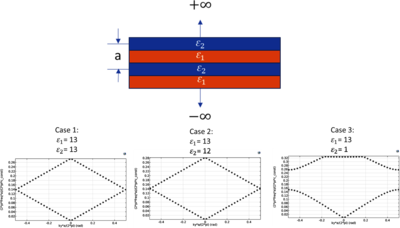# Application Gallery

## Three Cases for a 1D Photonic Crystal

Application ID: 89741

In this model, an eigenfrequency analysis is performed to give a bandgap analysis of a 1D multilayer photonic crystal extending to infinity in +/- y direction.

We perform the bandgap analysis for three different cases of material properties, as discussed in Chapter 4 of Ref. 1.

1. Case 1: epsr1 = 13 and epsr2 = 13
2. Case 2: epsr1 = 13 and epsr2 = 12
3. Case 3: epsr1 = 13 and epsr2 = 1

### Reference

1. J.D. Joannopoulus, R.D. Meade, and J.N. Winn, Photonic Crystals (Molding the Flow of Light), Princeton University Press, 2008.This model example illustrates applications of this type that would nominally be built using the following products: